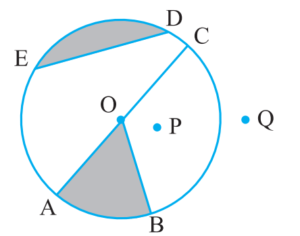# NCERT Class 6 Mathematics Fourth Chapter Basic Geometrical Ideas Exercise 4.6 Solutions

## EXERCISE 4.6

(1) From the figure, identify:(a) The centre of circle = OIn case you are missed :- Previous Chapter Exercise Solution

(e) Two points in the interior = O, P

(f) A point in the exterior = Q

(g) A sector = OAB

(h) A segment = Segment ED

(2) (a) Is every diameter of a circle also a chord? = Yes

(b) Is every chord of a circle also a diameter? = No

(3) Draw any circle and mark

(a) Its centre

(c) A diameter

(d) A sector

(e) A segment

(f) A point in its interior

(g) A point in its exterior

(h) An arc(4) Say true or false:

(a) Two diameters of a circle will necessarily intersect. = True

(b) The centre of a circle is always in its interior = True

In case you are missed :- Next Chapter Exercise Solution

Updated: April 14, 2023 — 1:54 pm